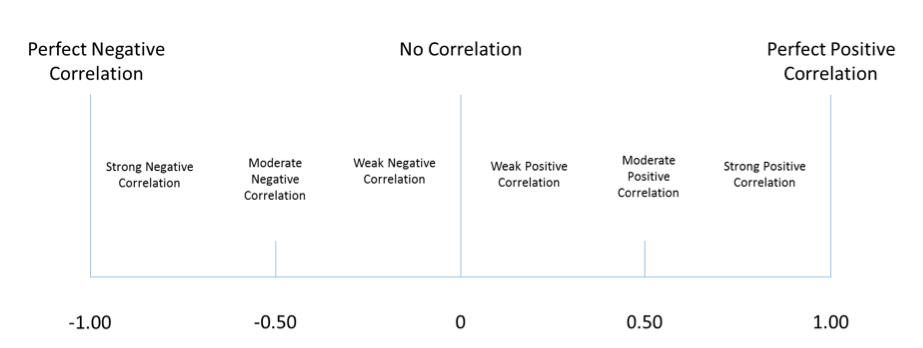Correlation between two variables is defined as the covariance of the two variables divided by the product of their standard deviations. The mathematical formula is presented below:Where,  is the covariance,   is the standard deviation of asset ,   is the mean of , and  is the expectation.

Properties of correlation of two random variables  and  are summarized here:

• Correlation measures the strength of the linear relationship between two random variables
• Correlation has no units
• The correlation ranges from -1 to +1
• If  the random variables have perfect positive correlation. This means that the movement in one random variable results in a proportional positive movement in the other relative to its mean.
• If  the random variables have perfect negative correlation. That means that a movement in one random variable results in an exact opposite proportional movement in the other relative to its mean.
• If  there is no linear relationship between the variables, indicating that prediction of  cannot be made on the basis of  using linear methods.For example, if the Correlation of trader PipMaster with the S&P500 is 0.87, we can say that there is a strong linear relationship, and if the S&P500 gains in value so would PipMaster’s account.

Another example, if we imagine that there are the only 10 year of returns available for PipMaster and the S&P500, from the example below we can say that there is a weak positive correlation. Or stated in plain terms, if the S&P500 is making positive returns, then also PipMaster will be making positive returns.